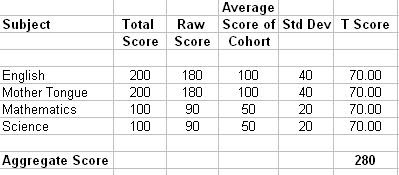This blog is managed by Song Hock Chye, author of Improve Your Thinking Skills in Maths (P1-P3 series), which is published and distributed by EPH.

## Saturday, November 24, 2007

### More on PSLE Aggregate and T-Scores

This post is Part 2 on the topic of PSLE Aggregate and T-Scores. In the last post in this blog , How PSLE Aggregate and T-Scores are calculated, we saw how those scores are calcuated.

One concern parents and students have is that there may be bias towards language subjects because the total score is 200, while the total for Maths and Science is 100.

In this post, we will see that there is no bias towards any particular subject, although the total scores for languages is twice that of Maths and Science.

Below is a tabulation to show that T-score eliminates any bias that may occur due to more total marks allocated to a particular subject.In the example above, the Raw Score of all subjects for this student is 90%.

English – 90% of 200 marks = 180 marks
MT - 90% of 200 marks = 180 marks
Maths – 90% of 100 marks = 90 marks
Science – 90% of 100 marks = 90 marks

The Average Score of the cohort for all subjects is 50% of the maximum score.

English – 50% of 200 marks = 100 marks
MT - 50% of 200 marks = 100 marks
Maths – 50% of 100 marks = 50 marks
Science – 50% of 100 marks = 50 marks

The Standard Deviation (Std Dev) is 40% from the average.

English – 40% of 100 marks (the average) = 40 marks
MT - 40% of 100 marks (the average) = 40 marks
Maths – 40% of 50 marks (the average) = 20 marks
Science – 40% of 50 marks (the average) = 20 marks

Using the T-score formula 50 + 10(X-Y)/Z, we can note that for all the subjects, the T-Score is 70. Although English and MT have a total score of 200, while Maths and Science have a total score of 100, this is automatically adjusted with the T-Score calculation.

There is no bias towards any subject in the calculation of PSLE Aggregate and T-Scores.

Parents and students need not be unduly worried.Anonymous said...

Thanks for the new info, but I still don't understand somethings:

1)For the cohort thing, is it the school's average or the whole Singapore's schools' average?

2)How do I estimate my aggregate when the teachers don't know the average?